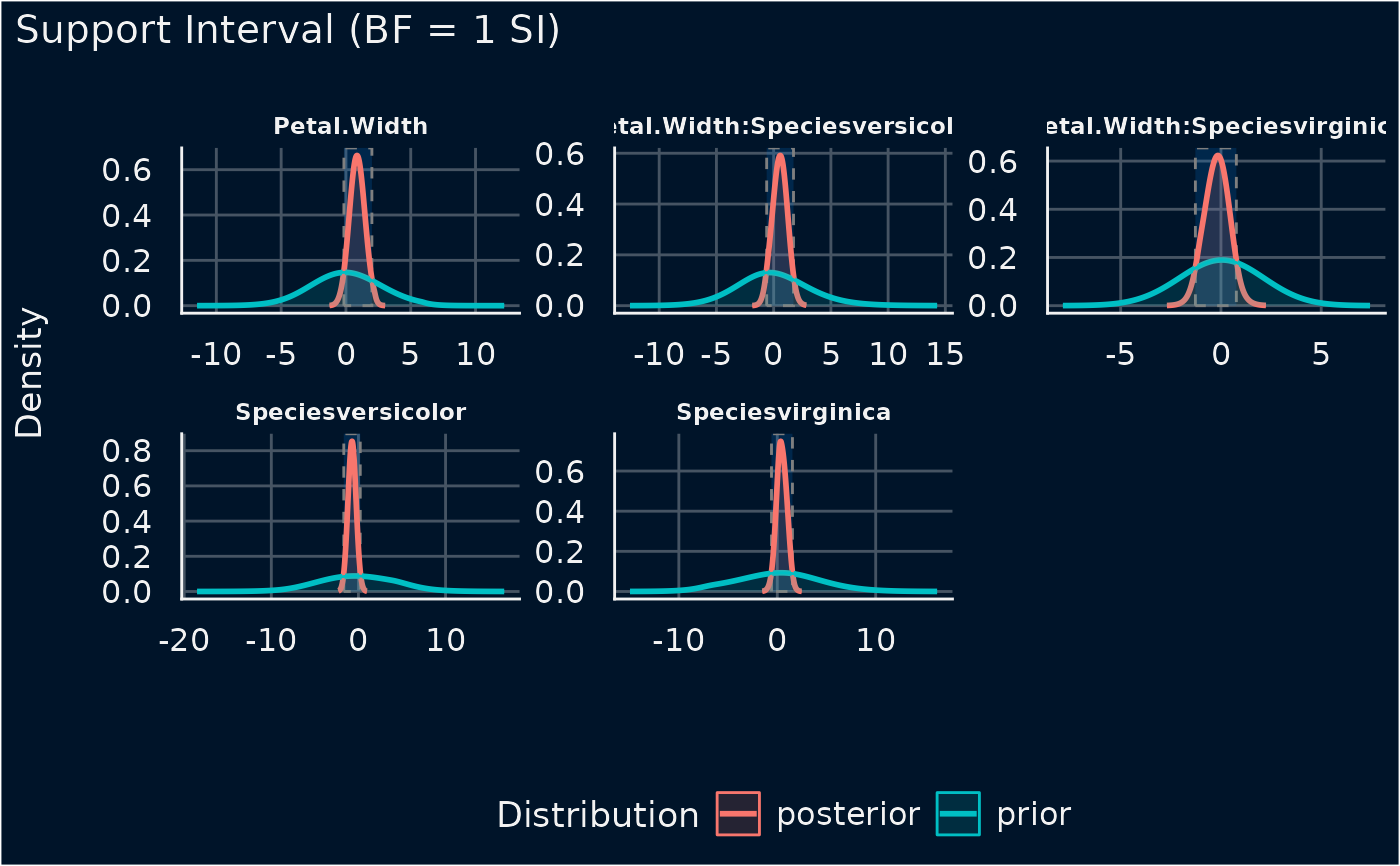The plot() method for the bayestestR::si().

## Usage

# S3 method for see_si
plot(
x,
si_color = "#0171D3",
si_alpha = 0.2,
show_intercept = FALSE,
support_only = FALSE,
...
)

## Arguments

x

An object.

si_color

Character specifying color of SI ribbon.

si_alpha

Numeric value specifying Transparency level of SI ribbon.

show_intercept

Logical, if TRUE, the intercept-parameter is included in the plot. By default, it is hidden because in many cases the intercept-parameter has a posterior distribution on a very different location, so density curves of posterior distributions for other parameters are hardly visible.

support_only

Logical. Decides whether to plot only the support data, or show the "raw" prior and posterior distributions? Only applies when plotting bayestestR::si().

...

Arguments passed to or from other methods.

## Value

A ggplot2-object.

## Examples

# \donttest{
if (require("bayestestR") && require("rstanarm")) {
set.seed(123)
m <- stan_glm(Sepal.Length ~ Petal.Width * Species, data = iris, refresh = 0)
result <- si(m)
result
plot(result)
}